# Assignments

1. Music and wine
Market researchers know that background music can influence the mood and purchasing behavior of customers. One study in a supermarket in Northern Ireland compared three treatments: no music, French accordion music, and Italian string music. Under each condition, the researchers recorded the numbers of bottles of French, Italian, and other wine purchased. Here is the contingency table that summarizes the data.

a. Formulate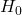and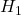.
b. Complete the table with the marginal totals of rows and columns and give the data.
c. The observed value in cell Other/None is 43. Compute the expected value of this cell.
d. An appropriate test statistic is the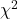-test. Give only the formula of this test.
e. It appears that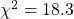. What is the critical value if the significance level is 5%.
f. Based on this information would you reject the null hypothesis?

Solution.
a.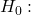Music and purchased wine are independent.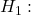They are not independent.
b. The row totals are 99; 31; 113. The column totals are 84; 75; 84.
c. The expected value of cell Other/None is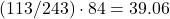.
d.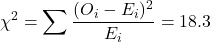.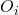are the observed values,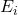are the expected values.
e. The significance level is 0.05 and the degrees of freedom are.  So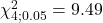.
f. Since the test statistic lies in the rejection region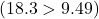the null hypothesis is rejected.

0